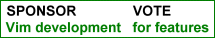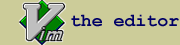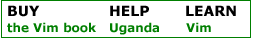SAS Indent : Indent Script for SAS 9.1/9.2/9.3/9.4

 created by Zhenhuan Hu script type indent description Indent script for SAS. The algorithm is supposed to be rather tolerable. Examples of the indenting results are shown as following: 1. For data step/proc sections, the script can properly indent with or without the closing run/quit statements.    (SAS does not require explicit closing statements for data step/proc sections.)    data female;       set total; where gender = 0;    data male;       set total; where gender = 1;    run;    proc sort data = new; by gender;    proc freq data = new;       table gender / missing;    endsas; 2. The script can also recognize which procedure supports run-processing and indent accordingly.    proc reg;       model y = x1-x5;    run;       model y = x1-x10;    run;    quit;       3. The indentation is properly managed when mixed with macros.    (Nesting macro is evil. Don't do it)    %macro test();       data new;          set old; where age >= 18;          format gender gender.;       proc freq;          table gender;    %mend; 4. Logic expressions.    data a;       set b; where age >= 18;       if numtx = 1 and not (pid > 0 and dcitag in (1, 2)) then strata = 3;       else if pid > 0 then do;          if dcitag in (1, 2) then do;             strata = 1; dcitype = 1;          end;          else if dcipsh1 = 1 then do;             strata = 1; dcitype = 3;          end;          else if numtx > 1 then strata = 2;       end;       else do;          if dcitag2 in (1, 2) then do; strata = 1; dcitype = 2; end;          else if dcipsh1 = 1 then do; strata = 1; dcitype = 3; end;          else if pid < 0 and dcipsh1 ~= 1 and numtx > 1 then strata = 2;       end;    run; Please also check out my SAS syntax script: http://vim.sourceforge.net/scripts/script.php?script_id=3522 install details

 rate this script Life Changing Helpful UnfulfillingIf you have questions or remarks about this site, visit the vimonline development pages. Please use this site responsibly. Questions about Vim should go to the maillist. Help Bram help Uganda. OSDN